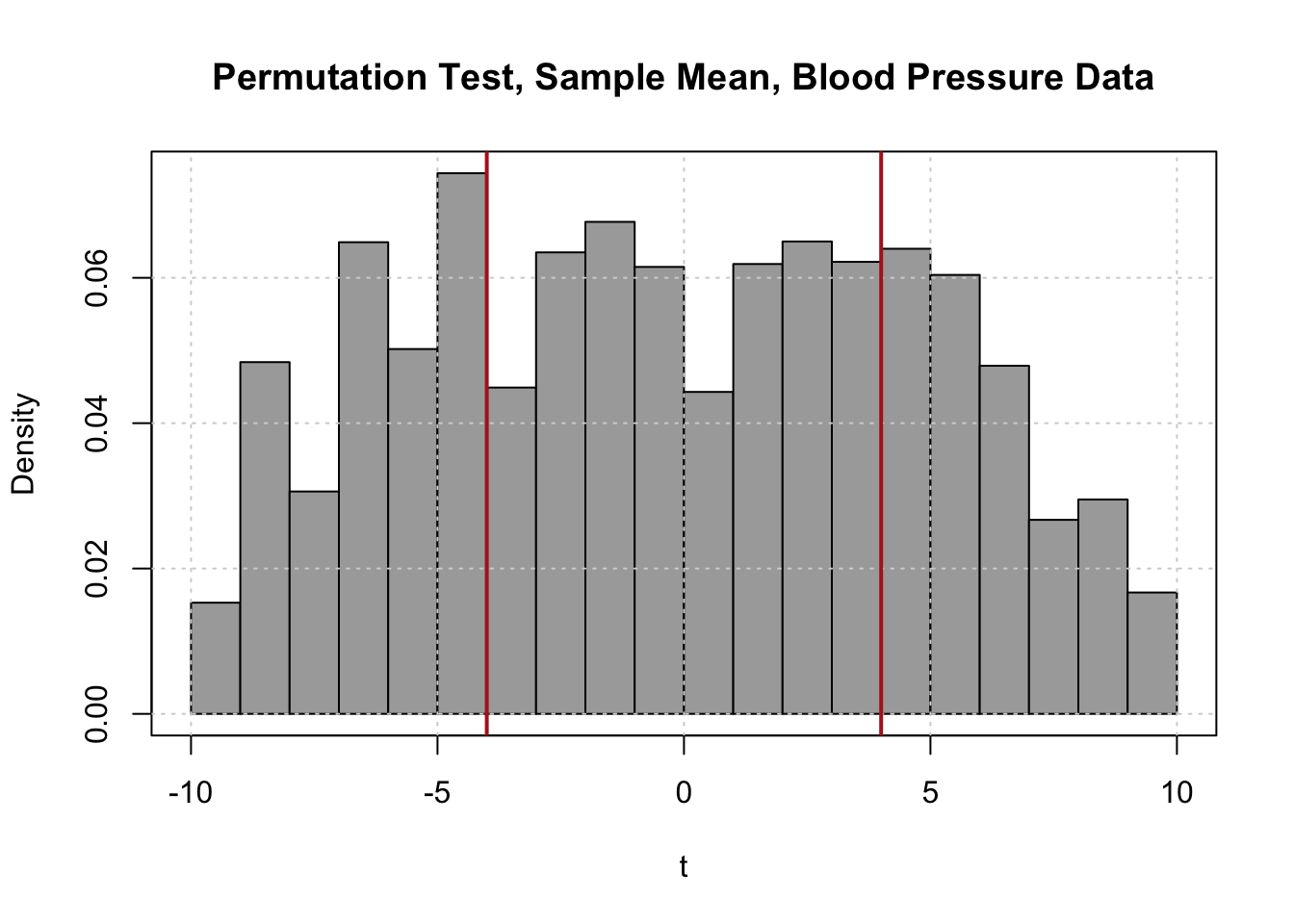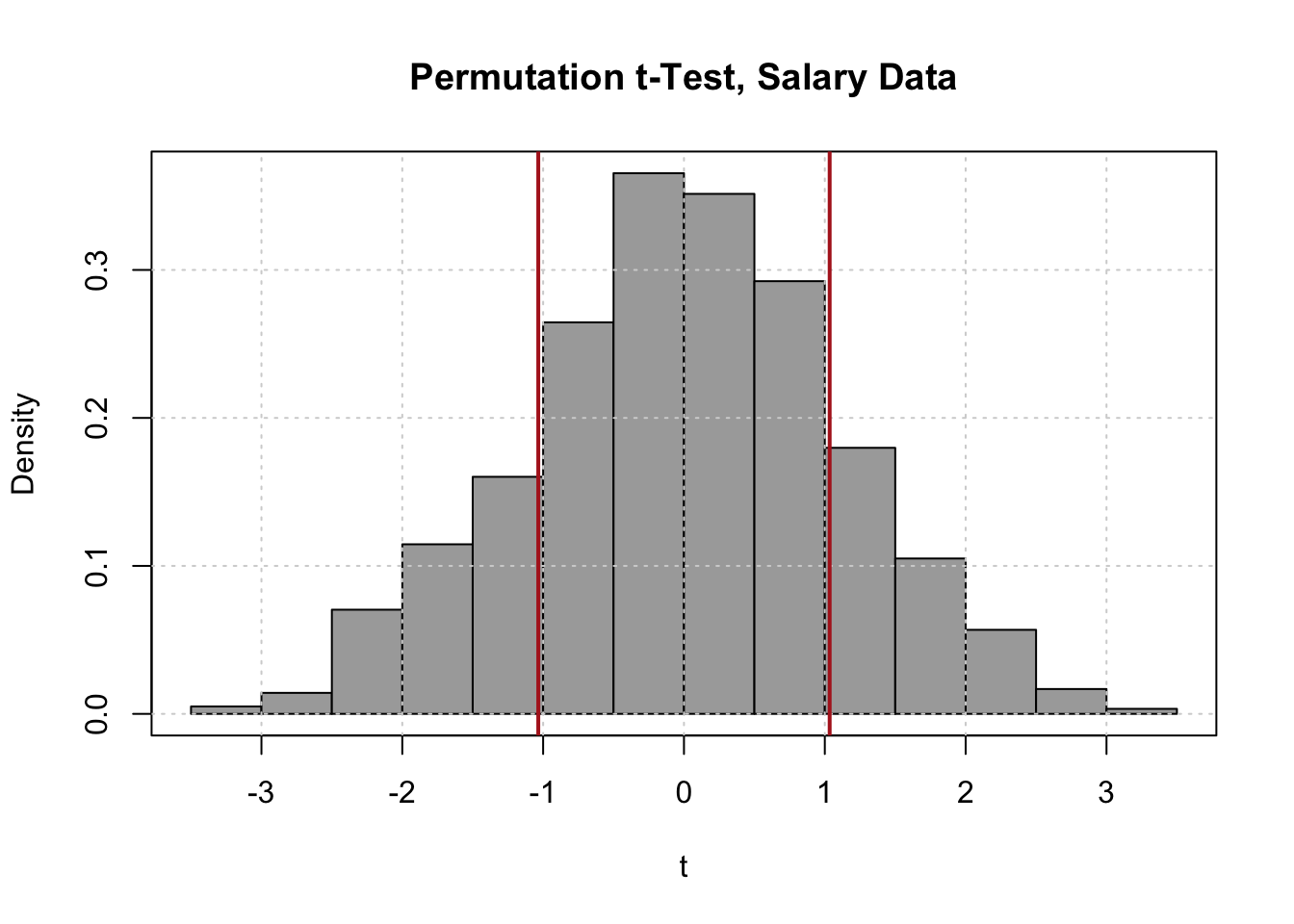# Exercise 1

Suppose that a researcher is interested in the effect of caffeine on typing speed. A group of nine individuals are administered a typing test. The following day, they repeat the typing test, this time after taking 400 mg of caffeine. (Note: This is not recommended.) The data gathered, measured in words per minute, is

decaf = c(98,  124, 107, 105, 80, 43, 73, 68, 69)
caff  = c(104, 128, 110, 108, 86, 53, 72, 73, 72)
decaf caff
98 104
124 128
107 110
105 108
80 86
43 53
73 72
68 73
69 72

Note that these are paired observations.

Use the sign test with a significance level of 0.05 to assess whether or not caffeine has an effect on typing speed. That is, test

$H_0\colon \ m_D = m_C - m_N = 0 \quad \text{vs} \quad H_A\colon \ m_D = m_C - m_N \neq 0$

where

• $$m_C$$ is the median typing speed in words per minute of individuals using caffeine
• $$m_N$$ is the median typing speed in words per minute of individuals not using caffeine

Since it is possible that the caffeine makes typing speed worse, use a two-sided test.

You may use the following probabilities calculated in R.

round(dbinom(x = 0:9, size = 9, prob = 0.5), 3)
##   0.002 0.018 0.070 0.164 0.246 0.246 0.164 0.070 0.018 0.002

Report:

• The p-value of the test
• A decision when $$\alpha = 0.05$$.

### Solution

# the "test statistic" for the sign test
sum(caff - decaf > 0)
##  8
# the expected value of the test stat under the null
# this is used to determine "extreme" values of the test statistic
length(caff - decaf) / 2
##  4.5
# add up the probabilities for test stat values that are as extreme or more extreme
sum(dbinom(c(0, 1, 8, 9), size = 9, prob = 0.5))
##  0.0390625
• P-Value: 0.0390625
• Decision: Reject $$H_0$$.

# Exercise 2

Does meditation have an effect on blood pressure. A group of six college aged individuals were given a routine physical examination including a measurement of their systolic blood pressure. (Measured in millimeters of mercury.) A week after their physicals, the same six individuals returned for a guided meditation session. Immediately afterwords there (systolic) blood pressure was measured. The data gathered is

physical    = c(125, 108, 185, 135, 112, 133)
meditation  = c(120, 114, 160, 131, 124, 125)
physical meditation
125 120
108 114
185 160
135 131
112 124
133 125

Note that these are paired observations.

Use the sign test with a significance level of 0.10 to assess whether or not meditation has an effect on blood pressure. That is, test

$H_0\colon \ m_D = m_M - m_P = 0 \quad \text{vs} \quad H_A\colon \ m_D = m_M - m_P \neq 0$

where

• $$m_P$$ is the median systolic blood pressure in millimeters of mercury measured without meditation
• $$m_M$$ is the median systolic blood pressure in millimeters of mercury measured with meditation

Since it is possible that the meditation makes blood pressure worse, use a two-sided test.

You may use the following probabilities calculated in R.

round(dbinom(x = 0:6, size = 6, prob = 0.5), 3)
##  0.016 0.094 0.234 0.312 0.234 0.094 0.016

Report:

• The p-value of the test
• A decision when $$\alpha = 0.10$$.

### Solution

# the "test statistic" for the sign test
sum(meditation - physical > 0)
##  2
# the expected value of the test stat under the null
# this is used to determine "extreme" values of the test statistic
length(meditation - physical) / 2
##  3
# add up the probabilities for test stat values that are as extreme or more extreme
sum(dbinom(c(0, 1, 2, 4, 5, 6), size = 6, prob = 0.5))
##  0.6875
• P-Value: 0.6875
• Decision: Fail to reject $$H_0$$.

# Exercise 3

• $$H_0$$: The distribution of systolic blood pressure is the same with and without meditation
• $$H_A$$: The distribution of systolic blood pressure is different with and without meditation

To do so, use a permutation test that permutes the statistic

$\bar{x}_D$

where $$\bar{x}_D$$ is the sample mean difference. Assume that the distribution of blood pressure with and without meditation has the same shape, but may have different locations. Use at least 10000 permutations.

physical    = c(125, 108, 185, 135, 112, 133)
meditation  = c(120, 114, 160, 131, 124, 125)
• Create a histogram that illustrates the distribution of the statistic used.
• Report the p-value of the test.

### Solution

# create difference data
bp_diff = meditation - physical

# function to shuffle data and calculate statistic
permute_x_bar = function(data) {
sample_size = length(data)
permuted_data = sample(c(-1, 1), size = sample_size, replace = TRUE) * data
mean(permuted_data)
}

# generate t statistics for sleep data
set.seed(42)
bp_x_bars = replicate(n = 10000, permute_x_bar(data = bp_diff))

# calculate t statistic on observed data
bp_x_bar_obs = mean(bp_diff)
hist(bp_x_bars, col = "darkgrey",
xlab = "t", probability = TRUE,
main = "Permutation Test, Sample Mean, Blood Pressure Data")
box()
grid()
abline(v = c(-1, 1) * bp_x_bar_obs, col = "firebrick", lwd = 2)mean(bp_x_bars > abs(bp_x_bar_obs)) + mean(bp_x_bars < -abs(bp_x_bar_obs))
##  0.5003
• This histogram looks real weird… This is mostly due to the choice of statistic to permute. Had we used some like the usual $$t$$ statistic, the histogram would be more normal looking.

# Example 4

Which profession pays more? Data Scientist of Actuary? A (far too small) survey of junior (less than three years experience) data scientist and actuaries resulted in the following data:

data_sci = c(88000, 121000, 91000, 50000, 78000, 95000)
actuary = c(63000, 75000, 81000, 75000, 85000)

Use a permutation test that permutes the statistic

$t = \frac{(\bar{x} - \bar{y}) - 0}{s_p\sqrt{\frac{1}{n_1} + \frac{1}{n_2}}}$

to test

• $$H_0$$: The distribution of salaries is the same for junior data scientists and actuaries
• $$H_A$$: The distribution of salaries is different for junior data scientists and actuaries

Assume that the distribution of salaries for both has the same shape, but may have different locations. Use at least 10000 permutations.

• Create a histogram that illustrates the distribution of the statistic used.
• Report the p-value of the test.

### Solution

# function to shuffle data and calculate statistic
permute_two_t_stat = function(data_1, data_2) {

# determine samples sizes of both groups
sample_size_1 = length(data_1)
sample_size_2 = length(data_2)

# create variable for group structure
groups = c(rep(TRUE, sample_size_1), rep(FALSE, sample_size_2))

# shuffle the groups
shuffled_groups = sample(groups)

# merge the data into a single group (null hypothesis)
all_data = c(data_1, data_2)

# create new groups
shuffled_data_1 = all_data[shuffled_groups]
shuffled_data_2 = all_data[!shuffled_groups]

# calculate statistics on permuted data
t.test(x = shuffled_data_1, y = shuffled_data_2, var.equal = TRUE)$statistic } # generate t statistics for exam data set.seed(42) salary_t_stats = replicate(n = 10000, permute_two_t_stat(data_1 = data_sci, data_2 = actuary)) # calculate t statistic on observed data salary_t_obs = t.test(x = data_sci, y = actuary, var.equal = TRUE)$statistic
hist(salary_t_stats, col = "darkgrey",
xlab = "t", probability = TRUE,
main = "Permutation t-Test, Salary Data")
box()
grid()
abline(v = c(-1, 1) * salary_t_obs, col = "firebrick", lwd = 2)mean(salary_t_stats > abs(salary_t_obs)) + mean(salary_t_stats < -abs(salary_t_obs))
##  0.3382

# Exercise 5

Repeat exercise 3, but use an appropriate test available in the R function wilcox.test().

Report:

• The p-value of the test
• A decision when $$\alpha = 0.05$$.

### Solution

wilcox.test(x = meditation, y = physical, paired = TRUE)
##
##  Wilcoxon signed rank test
##
## data:  meditation and physical
## V = 8, p-value = 0.6875
## alternative hypothesis: true location shift is not equal to 0
• P-Value: 0.6875
• Decision: Fail to reject $$H_0$$.

Here we used the Wilcoxon signed rank test as this is paired data.